## Wednesday, February 26, 2014

### Exceptions to Continuity 2

This is the second post of a three-part post. For the first, see here.

The question arose in the previous post whether for any set S in R, the real numbers, there exists a function f such that S is the set of discontinuities of f, or, in our previous notation, D(f) = S. Note that solving this problem is exactly equivalent to solving the problem of whether every set can be the set of points at which a function is continuous, since every function f is continuous at exactly the points that it is not discontinuous. Before tackling the whole problem, we solve it for a more limited class of functions, called the monotone functions.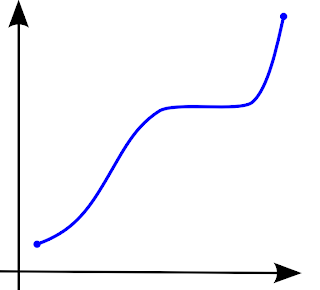A monotonically increasing function on an interval (image from wikipedia)
A function is monotone if it is either always increasing or always decreasing (such a function is called a monotonically increasing or monotonically decreasing function). A function f is increasing if for any real numbers a and b, ab means that f(a) ≤ f(b), with a similar definition for decreasing. The function above increases, then stays constant for awhile, and then continues to increase. It is thus a monotonically increasing function. Some common examples of monotonically increasing functions are y = x, y = c (for any constant c), y = xn (where n is an odd integer), and y = ex.

Next, it is important to note that there is a one-to-one correspondence between monotonically increasing and monotonically decreasing functions caused by flipping sign, that is, the negative of a monotonically increasing function is monotonically decreasing, and the negative of every monotonically decreasing function is monotonically increasing. Thus y = -x, etc., are monotonically decreasing.

A theorem in mathematics called Froda's theorem after the Romanian mathematician Alexandru Froda completely classifies the possible sets of discontinuities over the monotone functions. We outline the proof of the theorem, noting that, due to the one-to-one correspondence discussed above, the result for monotonically increasing functions will hold for all monotone functions. The statement runs thus: For any monotone function f defined on closed interval [a,b], D(f) is at most a countable set in [a,b], that is, the set is empty (for an everywhere continuous function), finite, or countable (can be put in a one-to-one correspondence with the natural numbers).

The first step in the proof is noting that, for a monotonically increasing function f defined on the interval [a,b], the only discontinuities that f can have are jump discontinuities. Other types of continuities require some type of infinite oscillation (e.g. the function y = sin(1/x)), but this involves the function increasing and decreasing, which is not allowed for monotone functions. Thus we consider jump discontinuities only.

Consider the difference d = f(b) - f(a) of the values of the function at the endpoints of the interval [a,b]. Clearly it is positive and finite, due to the properties of f and the fact that it is defined on both endpoints. Note also that the jumps can only be positive, since the function is increasing; the function can only jump up, not down. For any positive number ε, therefore, there can only be a finite number of jumps of length ε, since the sum of the jumps cannot exceed d.

For example, in the above diagram, the function f, which increases from 1 to 3 over the interval [a,b], cannot have more than two jump discontinuities with jump 1, since the sum would then exceed the value of d, in this case, 2.

Therefore, given a monotonically increasing function f, for each positive number ε we can construct a set of points {x1,x2,...,xn}, containing every point that is a jump discontinuity of f with a jump greater than or equal to ε. By the previous remarks, this set is finite for any positive ε (although it is allowed to be empty, e.g., for continuous monotonically increasing functions). Finally, we define Dn(f) for a positive integer n to be the set corresponding to the value ε = 1/n. The union of all of the Dn for positive integer n then includes every jump discontinuity of f, since for any jump discontinuity with jump ε, ε is greater than 1/n for some n. If the jump were 0, the discontinuity would not be a discontinuity at all! Thus every jump discontinuity is in some Dn, and thus in their union, which we shall call D, as consistent with previous notation.

Therefore, the set of discontinuities of a monotonically increasing function f (and similarly for all monotone functions) is a countable union of finite sets, which is countable.

This result is extensible to a monotone function on the real numbers. The set of real numbers R can be expressed as a countable union of closed intervals [n,n + 1], where the union is over every integer n. One can simply collect the discontinuities from each interval (on which the function must be monotone, since it is monotone everywhere) into a larger set. Since a countable union of countable sets is countable, the set of discontinuities over all of R is countable also. Lastly, this result also extends to any function f defined on the real numbers such that there is a countable collection of sets A such that the union of all members of A is R and f is monotone on each member of A. In other words, if the real numbers can be appropriately split into pieces on which a function f is monotone, then the set of discontinuities of f is countable.

As general as this result may be, it certainly does not classify the sets of discontinuities of all functions. We have already seen an example of a function discontinuous everywhere. The function of that example is not monotone on any interval, so Froda's theorem is inapplicable.

To complete the classification of sets of discontinuities, we must introduce the notion of a Fσ set. A subset S of the real numbers is an Fσ set if and only if it is a countable union of closed sets. A closed set in the context of the real numbers is one which contains its boundary points. For example, [0,1], the interval containing 0 and 1, is a closed interval, because it contains its boundary points, 0 and 1. The sets (0,1), [0,1), and (0,1], missing one or both endpoints, are not closed. In addition, any union of disjoint closed intervals such as [-1,2]∪[3,4]∪[5,7] is closed.

The realm of Fσ sets is quite general. First, since R itself, the set of real numbers, has no boundary, it by definition must "contain" its boundary, and is thus closed, and therefore Fσ. Since the empty set has no boundary, it is also closed, and {} is Fσ. Any countable set of points is also Fσ, as a set containing one point, {x}, is always closed, and the collection of Fσ sets is closed under countable union. Thus Q is Fσ. Finally, as an example of a set that is not Fσ, consider the set of irrationals, R - Q. Since Q is dense, i.e. there is a rational number between any two distinct real numbers, no part of R - Q has an interior; there are no "blocks" of irrational numbers with no intervening rationals among the reals. Thus to get the irrationals into a collection of closed sets, we have to "package" them individually, using, for example, countable collections of points. However, the irrationals are uncountable, so no countable union of closed sets can yield R - Q. With this definition, we state the following theorem completely classifying sets of discontinuities:

A set S can occur as a set of discontinuities of a function f on the real numbers if and only if S is Fσ.

The proof that every function f has D(f) an Fσ set is too difficult to consider here. However, we can demonstrate the proof in the reverse direction. To finish this series (see the next post), we construct a function whose discontinuities form an arbitrarily chosen Fσ set.

Sources: Counterexamples in Analysis by Bernard R. Gelbaum and John M. H. Olmsted, Froda's Theorem on Wikipedia, http://holdenlee.wordpress.com/2010/04/26/can-a-function-be-continuous-only-on-rationals/, http://samjshah.com/2009/10/03/sin1x/,

## Tuesday, February 18, 2014

### Exceptions to Continuity 1

An important class of functions in mathematics is the class of continuous functions. A continuous function is a function without any jumps or undefined points (for a more formal definition, see here). For convenience, we shall limit our scope to functions f on the real numbers.

The class of continuous functions on the real numbers is quite general; all linear, polynomial, and exponential functions fall under this category. However, many other functions fall into the class of discontinuous functions, or those which have at least one point at which they are not continuous. Take as an example the Heaviside step function, which is (conditionally) defined by the equation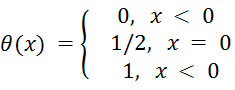.

This function, often denoted θ(x) as above, is graphed below.The function assumes a constant value of 0 as the value of x approaches 0, and then at zero, it assumes the value 1/2 (solid blue dot). θ(x) then equals 1 for all positive values of x. This function is discontinuous at exactly one point, namely at x = 0 because the function jumps from 0 to 1/2 to 1 at this point. We can then consider the set of points of discontinuity of the function, which for a function f we shall denote D(f) (where "D" stands for discontinuity). In this way, D itself is a function; to each function f on the real numbers it associates the set of points of discontinuity of f. For instance, if f(x) = x2, then D(f) = Ø (the empty set), and, by the discussion above, D(θ) = {0}.

However, many functions have more than one point of discontinuity. Some in fact have an infinite number of discontinuities! Take for example the greatest integer or floor function, which to every real number assigns the greatest integer less than or equal to it. For example, floor(2.5) = 2, floor(6.93) = 6, and floor(1) = 1. The graph of this function is shown below: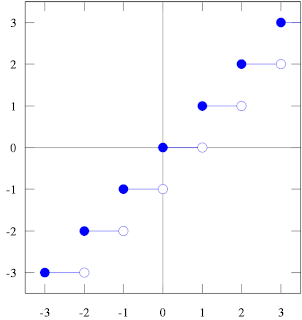The set of discontinuities of the floor function is just the set of integers; as soon as the input of the function increases from 0.999 to 1.000, for example, the output jumps from 0 to 1. Thus D(floor) = Z = {...-3,-2,-1,0,1,2,3,...} (Z is often used to stand for the set of integers). Even for this function, however, the discontiuities are isolated, and "most" of the function still behaves normally. However, there are functions for which every real number is a discontinuity. One notable example is the Dirichlet function, denoted IQ(x) and defined on all real numbers as being equal to 1 if x is rational, and 0 if it is not (see here for information on and examples of rational and irrational numbers). The function is denoted this way because it is also called the characteristic function of the rational numbers on the reals. A characteristic function of any set is the function that is equal to 1 on the set and 0 elsewhere. The symbol IQ indicates that the set in question is Q, the rational numbers. It is difficult to imagine the graph of this function, but it consists of an (countably) infinite set of points along the line y = 1 and an (uncountably) infinite set of points along the line y = 0.

To see that IQ(x) is discontinuous at every real number x, consider the following argument. If x is irrational, let the decimal expansion of x be 0.a1a2a3..., where a1, a2, a3, etc. are the digits of the infinite expansion. Clearly, every member of the sequence 0.a1000..., 0.a1a200..., 0.a1a2a30... is a rational number (as the decimal expansion of each terminates) and the sequence generates rational numbers arbitrarily close to the irrational number x. Thus the function takes the value 1 at points arbitrarily close to where it takes the value 0 and IQ(x) is discontinuous at x. For the case in which x is rational, it is clear that there is an irrational number between every two rationals (for definiteness, one such an irrational number between x and y would be (2-1/2)x + (1 - 2-1/2)y), so the rationals must be isolated points, and thus discontinuities of the function. In conclusion, the function IQ(x), though defined at every real number, is discontinuous at every point in its domain, so D(IQ) = R, the set of real numbers.

Though one might argue that the odd definition of this function seems artificial, as it uses rational and irrational numbers in its definition, this particular function does have a closed form, albeit in the form of an infinite limit. The function IQ(x) can also be defined by the expression:In the expression above, the limit is calculated pointwise, i.e. the value of the limit is calculated for each real number x, giving the value of the function at x. To see that this expression indeed produces the characteristic function of the rational numbers on the real numbers, first consider the inner limit, indexed by the variable j, for constant k (assume k = 1, so k! = 1). The function cos(πx) is equal to 1 or -1 at every integer.For large j, cos(πx)2j = 1 at all integers. The "2" in the exponent insures that all outputs of the function are positive. If the exponent were just j, the value of the function at x = 1 would simply oscillate between -1 and 1 and never converge, and the function would be undefined at odd integers. For any point that is not an integer, the value of cos(πx) will have an absolute value less than 1 and therefore approach zero in the limit. The inner limit therefore gives a function which has the value 1 at every integer and 0 elsewhere.

Now let k→+∞. We have already seen that the function will have value 1 whenever the expression within the cosine is integral. For any rational number r/q, where r and q are integers and the fraction is reduced to lowest form, clearly q! (q factorial) has q as a factor. Thus q!(r/q) is an integer. Finally, since q! is a factor of every subsequent factorial, k!(r/q) is an integer for every kq. Therefore, the value of the limit at every rational number becomes 1 as k increases without bound. If the function were 1 at an irrational number s, however, we would have k!(s) equal to an integer for some k, because integers are the only values for which cos(πx) = 1. Thus s would be expressible as a quotient of two integers, which is a contradiction, since s is irrational. We have then confirmed that the function expression indeed gives IQ(x).

Another important example is a modified version of Dirichlet's function, called Thomae's function or the popcorn function. It is defined in the following way.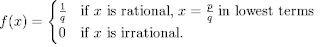This function agrees with Dirichlet's function on the irrational numbers, but for any rational number gives the reciprocal of its denominator in lowest form. At the nonzero integers, since these numbers can be represented as n/1, the function assumes the value 1. For simplicity, we also assume that f(0) = 1. A graph of this function is shown below (click to enlarge):The above shows a graph (a collection of points, in this case) of Thomae's function on the interval (0,1), and some examples of points: 1/2 yields the largest possible output of any number between 0 and 1, namely 1/2. Also, along the horizontal line y = 1/q for any positive integer n lie all points (p/q,1/q), where p and q share no factors, or are said to be relatively prime. Thus the number of points along each line y = 1/q also gives the number of positive integers less than or equal to q that are relatively prime to q. For example, the point (2/5,1/5) is illustrated, and there are a total of four points along the horizontal line containing it, since 1, 2, 3, and 4 are all relatively prime to 5. Notice however that (2/4,1/4) is not a valid ordered pair satisfying Thomae's function because 2/4 can be simplified to 1/2. The points get denser as one approaches the x-axis, (for example following the sequence of points (1/n,1/n), and the only horizontal line along which there are an infinite number of points is y = 0, since Thomae's function assumes the value 0 at every irrational number.

Now we must consider where Thomae's function, if anywhere, is continuous. Clearly, it is discontinuous at any rational number for the same reason as before: any rational number yields a nonzero output, but is surrounded by irrational numbers which are mapped to 0. However, using the formal definition of continuity, we can confirm that Thomae's function is actually continuous on the irrational numbers. The function will be continuous there if the differences in the outputs at an irrational number and at "nearby" points tend to 0 as the "nearby" points come closer and closer to the chosen number. Let s be any irrational, and choose any small positive number ε to be the desired upper bound for the difference between the value of the function at s and at a nearby point. Let q be the smallest positive integer such that ε > 1/q. Since s is irrational, clearly it is not equal to any p/q (p,q relatively prime). Let δ be the distance between s and the closest rational number (in lowest form) with a denominator less than or equal to q. Within a distance δ of s, the function assumes values strictly less than 1/q. Thus the largest possible difference is less than 1/q (since f(s) = 0) which is less than ε, and the function is continuous at s. To make this clearer, the above reasoning is made explicit in the diagram below (click to enlarge):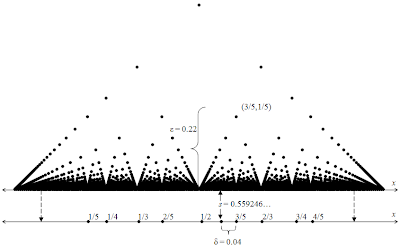A demonstration of the above reasoning for an arbitrary irrational s = 0.559246... and ε = 0.22
In the above diagram, the smallest positive integer with ε > 1/q is q = 5, since 1/5 = 0.20 < 0.22. Among those rationals with denominators less than or equal to 5 (which are copied on a duplicated x-axis so that they can more easily be seen), 3/5 is the one closest to s, with a difference δ of about 0.04. If, for any x, x is within a distance 0.04 of s, the function value at x is guaranteed to be less than 0.20 and so within 0.22 of the value of the function at s, 0. Since this can be done for any positive ε, the function is continuous at s. Similarly, the function is continuous at every irrational.

Therefore, there exists a function f (Thomae's function) with D(f) = Q (the set of rational numbers). The question naturally arises whether any set can be the set of discontinuities of a function. This question is explored in the next post.

Sources: Counterexamples in Analysis by Bernard R. Gelbaum and John M. H. Olmsted, The Road to Reality by Roger Penrose, Weierstrass Function, Dirichlet Function on Wikipedia, http://www.maa.org/pubs/Calc_articles/ma001.pdf, http://www.whitman.edu/mathematics/SeniorProjectArchive/2007/huh.pdf

## Monday, February 10, 2014

### Further Properties of Space-filling Curves

This is the final part of a three-part post. For the first, see here.

In the previous post, we examined an iterative sequence of curves, the limit of which after an infinite number of iterations is called the Hilbert curve. We now prove that the Hilbert curve indeed has the property it was constructed to have: it fills the unit square. Consider the first iteration again.The first iteration includes all four points of the form (m/2,n/2) when m and n are each either one or three. In binary decimal notation, 1/4 = 0.01 and 3/4 = 0.11. To see the relevance of this fact, now consider the second iteration.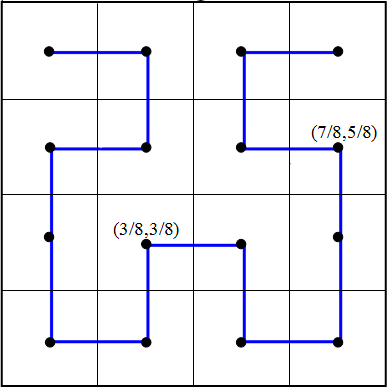The points along the curve marked by black dots are of the form (m/8,n/8) with m and n both odd (and between 0 and 8), and two of these points are explicitly marked with their coordinates for clarity. It is obvious that the second iteration contains all points of this form. In binary notation, the points are of the form (0.ab1,0.cd1), where a, b, c, and d can stand for either 0 or 1. For example, (7/8,5/8) = (0.111,0.101). Since each iteration contains smaller copies of the previous one, it is clear that this pattern continues, and the third iteration will include all points of the form (m/16,n/16), with 0 < m, n < 16 and both m and n odd. In decimal notation, the curve includes all points each of whose coordinates has a 4-digit terminating binary expansion that ends in 1 (just as the second iteration had 3-digit terminating binary expansions ending in 1).

Next, we must note that any binary expansion can be approximated to arbitrary accuracy by others ending in the digit "1". For example, let P be the point in the unit square (0.101010101...,0.10100000...), where the repetition in the first expansion continues infinitely. Now consider the sequence {(0.11,0.11), (0.101,0.101),(0.1011, 0.1011),(0.10101,0.10001),...}. The nth point in this sequence lies on the curve of the nth iteration, and the sequence converges to the point P defined above. Thus the point P lies on the limit of the iterations, i.e., the Hilbert Curve itself. Note that the second coordinate of point P is exactly matched by the point on the 2nd iteration, and the sequence goes on to actually veer away (temporarily) from its final value. However, it still approaches the same limit as the sequence continues.

Since binary is simply another way of writing the real numbers, it is easy to show that there is a binary expansion for every real number from 0 to 1. For more discussion on this topic, see The Infinity Series, specifically here. Therefore, the Hilbert Curve visits every point in (and on) the unit square, and is indeed a space-filling curve. We note next that the Hilbert curve actually "overachieves" its goal; it actually visits several points of the unit square multiple times. For example, the point (1/2,1/2) is the limit of the sequence {(0.11,0.11), (0.101,0.101),(0.1001, 0.1001),(0.10001,0.10001),...} as well as of another sequence {(0.01,0.01), (0.011,0.011),(0.0111, 0.0111),(0.01111,0.01111),...}. Thus the function mapping the unit interval onto the Hilbert Curve is not one-to-one though it is continuous. Contrast this with the first function we constructed from the unit interval onto the unit square, which was one-to-one (after resolving the issue of multiple decimal representations) but not continuous.

It is impossible for a function from the unit interval to the unit square to be one-to-one and continuous. Such functions are called homeomorphisms, and two spaces for which is it possible to construct a homeomorphism mapping one onto the other are topologically equivalent in a certain sense. This means that they share certain intrinsic toplogical properties. For example, if a homeomorphism from a space that is connected (see image below) to another space exists, the latter space must also be connected.Examples of connected and disconnected spaces.    Connected spaces can only have one component (A),  while  disconnected ones have multiple disjoint components (B).

Also, two homeomorphic spaces (this means that there is a homeomorphism from one onto the other) share other connectedness properties. For example, the unit interval has the property that, when any point of it (except an endpoint) is removed, it becomes disconnected. However, the unit square cannot be disconnected by the removal of any single point. Hence the two spaces differ in a topological property that is conserved by homeomorphisms and are not homeomorphic. Therefore, a continuous space-filling curve that is also one-to-one is impossible.

It is also worth noting that the concept of a Hilbert curve can be extended to any finite dimension; in three dimensions, instead of filling the unit square, the curve fills the unit cube. This would be done by dividing the cube into 23 = 8 cubes of side length 1/2 and divding the unit interval into 8 corresponding subintervals for the first iteration, and so on. Generally, the Hilbert curve, like many other mathematical oddities, shatters our intuition when it comes to what properties mathematical objects can possess, and reminds us of the differences between the physical and mathematical worlds.

Sources: Counterexamples in Analysis by Bernard R. Gelbaum and John M. H. Olmsted, Space-filling Curve on Wikipedia, http://upload.wikimedia.org/wikipedia/commons/9/95/Connected_and_disconnected_spaces.svg

## Sunday, February 2, 2014

### Constructing a Space-filling Curve

This is the second part of a three-part post. For the first, see here.

The previous post introduced the concept of a curve in the plane, presenting it as a type of mapping from one-dimensional to two-dimensional space, with the added condition that it be continuous. Finally, an interesting mapping that fills the unit square was presented. However, this example is not continuous. To show this, we shall demonstrate that a particular sequence {xn} = {x1,x2,x3,...} of points in t-space (the one-dimensional domain of the mapping) converges to a point x, also in the domain of f. Then, we shall show that the corresponding sequence of points {f(x1),f(x2),f(x3),...} does not converge to the image f(x) of the limit of the sequence. This will prove that the mapping is discontinuous.

Let {xn} be the sequence {0.490000...,0.499000...,0.499900...,...}. Clearly this sequence converges to x = 0.500. The sequence in the unit square {(0.400...,0.900...),(0.490...,0.900...),(0.490...,0.990...)}, whose members correspond to the value of f at each member of {xn} converges to (0.500...,0.999...), but the value of f at x is not this value. Rather it is f(0.500...) = (0.500...,0.000...). Thus f is not a continuous mapping. Note that even if the non-terminating decimal expansions were selected for those members of the unit interval where there are two possibilities, the function would still be discontinuous, and one would this time use a sequence of points approaching t = 1/2 from above rather than below.

Though, as mentioned previously, Peano was the first to demonstrate the existence of a continuous space-filling curve, the process described below actually refers to a space-filling curve devised by Hilbert shortly afterward, as it is more straightforward geometrically.

To actually construct a space-filling curve f that is continuous, we make use of a limiting process, which goes through steps called iterations. The first iteration of the process that results in the curve is as shown below: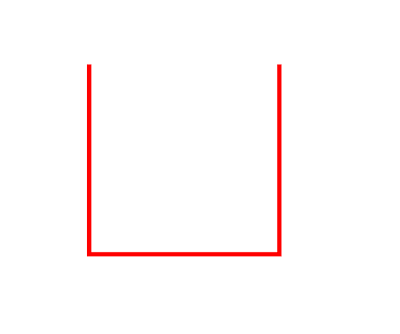This is the image of the first iteration of the mapping in the plane, but the way it is constructed is more easily understood when the square is divided into four smaller squares. The part of the curve lying in each smaller square is the image of one-quarter of the unit interval, as illustrated below.Each subset of the unit interval marked with a Roman numeral is mapped to the corresponding red segment contained within the square marked by the same numeral. Clearly the parametrization of the red curve defined this way is continuous, and is a mapping of the unit interval into the unit square. Also, at each point, the segment is equidistant from the center and edge of the square, so each of the three straight parts is of length 1/2.

The next step is to apply the limiting process. The second iteration of the curve is essentially several smaller connected copies of the first, as shown below.The second iteration of the limiting process (the blue curve at bottom) consists of four copies of the first iteration (some have been rotated) and segments connecting them so that the curve is continuous. Instead of mapping 1/4 of the unit interval into the segment contained within each square we now cut the interval into 16 regions and map each to the part of the curve within each of the 16 squares of side length 1/4 in the proper order. The first eight squares are numbered by Roman numerals in the diagram.

The process then continues, with each step consisting of four copies of the previous one, rotated in the same manner. On the nth step of the process, we can visualize the mapping as taking each subset of the unit interval of length 1/4n to the portion of the curve contained within a square of side length 1/2n, of which there are 4n in total.

Note in addition that the curve of the first iteration is composed of three segments of length 1/2, and thus has total length 3/2. Since the second iteration is made up of copies of the first scaled down by a factor of 1/2 (to fit in the squares which have half the sidelength of the original), it is made of 12 segments of length 1/4, plus 3 additional segments of this length to connect the copies. Thus the total length of the curve of the second iteration is 15/4. Generally, it is easy to see that, taking into account the segments that must be attached to the copies of previous iterations to connect them, that the length of the nth iteration is 2n - 1/2n. This sequence of lengths increases without bound, and therefore the end result, the Hilbert curve, is a curve of infinite length contained within a finite area.

In the next post, we prove that the Hilbert curve indeed fills the unit square and explore more of its properties and extensions.

Counterexamples in Analysis by Bernard R. Gelbaum and John M. H. Olmsted, Hilbert Curve on Wikipedia